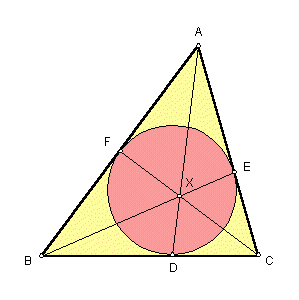# GERGONNE POINT

 In the plane of any triangle ABC, let D = point where the incircle meets side BC, E = point where the incircle meets side CA, F = point where the incircle meets side AB. The lines AD, BE, CF meet in a point, labeled X in the figure. It is called the Gergonne point of triangle ABC.Triangle Centers